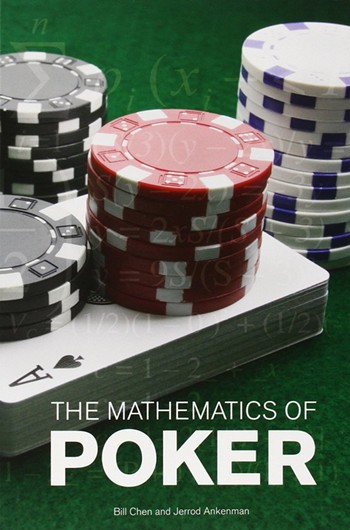# 【6upoker】扑克中的数学-7：EV（上）

• A+

《The Mathematics of Poker》中文翻译当一个概率分布的每一个可能出现的结果都对应一个数值型的收益时，我们可以求出这个概率分布的期望收益（EV），也就是将每一个收益分别乘以它们出现的概率，并将其加总。在这本书中，我们用<X>表示X的EV。举个例子：

PokerStars亚洲版(PS281.COM)全球最大德州扑克平台。发牌公正，与世界玩家同台竞技

《The Mathematics of Poker》中文翻译<B>=(1/2)*(+\$10)+(1/2)*(-\$10)=\$5+(-\$5)=0

<P>==p1x1+p2x2+......pnxn

<Bn>=(1/2)*(+\$11)+(1/2)*(-\$10)=\$5.5+(-\$5)=\$0.5

<Bd>=(1/36)*(+\$30)+(35/36)*(-\$1)=\$30/36+(-\$35/36)=-\$5/36，大约是-14美分。

+1

6UP-德扑第一平台&PokerStars亚洲唯一合作伙伴,新会员首存100送50元.

6UP扑克之星官网发布页：www.6updh.com